Our World Statistics Day conversations have been a great reminder of how much statistics can inform our lives. Do you have an example of how statistics has made a difference in your life? Share your story with the Community!
Choose Language Hide Translation Bar
When is a column not a column?

Scripting JMP often involves using data column objects. We might want to compute statistics for a column of numeric data. We might want to change a column attribute or property. In any of these cases, we need a reference to the column so that we can provide it as an argument. There are several ways to get or use a reference. Unfortunately, they might seem to be equivalent up to a point until we are surprised. A scientific theory is testable. A theory holds until a test invalidates it. Let's test some theories about column references.

I am using the height data column in the Big Class data table from the JMP sample data folder, which is C:\Program Files\SAS\JMPPRO\15\Samples\Data in my case. Let's get started.

``````Names Default To Here( 1 );

// open a data table
dt = Open( "\$SAMPLE_DATA/Big Class.jmp" );``````

Our first test will use several different forms of a column reference for height.

``````// use a reference to a data column
sum = Col Sum( :height );
Show( sum );
sum = Col Sum( Column( "height" ) );
Show( sum );
sum = Col Sum( Column( 4 ) );
Show( sum );
sum = Col Sum( :Name( "height" ) );
Show( sum );
sum = Col Sum( As Column( "height" ) );
Show( sum );``````

OK, what did we get?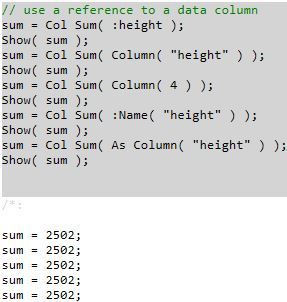Same result every time. So my theory is that these five different references are equivalent. My first test confirms my theory. But it is only the first test. Let's devise another test. Let's first get the column reference, store it, then use the stored reference to compute the same statistic.

``````// get a reference to a data column and then use it
col = Column( "height" );
sum = Col Sum( col );
Show( sum );
col = Column( 4 );
sum = Col Sum( col );
Show( sum );
col = :Name( "height" );
sum = Col Sum( col );
Show( sum );
col = As Column( "height" );
sum = Col Sum( col );
Show( sum );``````

Note that I did not include the very first form of a reference, :height because the value returned and stored in the expression col = :height is missing (".")! That result is not helping my theory! What did we get with the rest of test?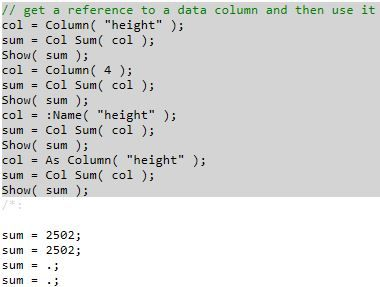Wait a minute! I didn't get a single error, but I didn't get the same result each time. The first two results are correct, but the last two are wrong. Let's perform one more test of my theory. Let's again obtain a column reference, store it, and then use it to send a message.

``````// get a reference to a data column and then use it
col = Column( "height" );
type  = col << Get Data Type;
Show( type );
col = Column( 4 );
type  = col << Get Data Type;
Show( type );
col = :Name( "height" );
type  = col << Get Data Type;
Show( type );
col = As Column( "height" );
type  = col << Get Data Type;
Show( type );``````

Here is what I get with the first two ways.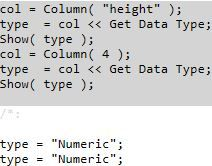The first two ways seem to work as expected. Let's try the third way.

``````col = :Name( "height" );
type  = col << Get Data Type;
Show( type );``````

This way didn't work.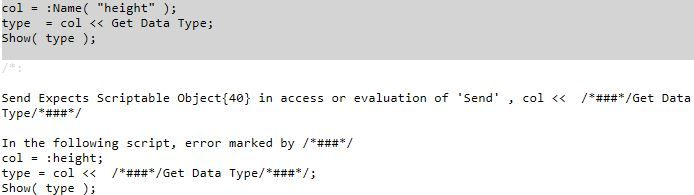``````col = As Column( "height" );
type  = col << Get Data Type;
Show( type );``````

I get the same error as the last time.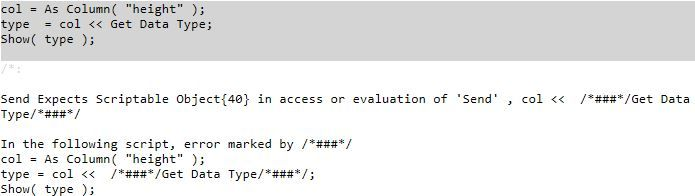It is clearly time to revise my theory. (And maybe to confide in the JMP developers.) My theory is now that

• :height, Column( "height" ), Column( 4 ), :Name( "height" ), and As Column( "height" ) all work or act as valid a column reference.
• Column( "height" ) and Column( 4 ) are functions that return a column reference.
• :height, :Name( "height" ), and As Column( "height" ) are not functions that return a column reference.
• As Column() is an alias for the colon (":") scoping operator, as seen in :height, which both return the value of the current row if used in an assignment.

So what is my recommendation?

• Use :height, Column( "height" ), Column( 4 ), :Name( "height" ), or As Column( "height" )  as a column reference.
• Use Column( "height" ) or Column( 4 ) functions to get a column reference.
• Do not use :height, :Name( "height" ), or As Column( "height" ) to get a column reference.

I hope that this adaptation (abomination?) of the scientific method helped to clarify how column references can be used in JMP scripting. I hope that you follow the recommendations and avoid further confusion and frustration.

Article Labels
Article TagsSuper User

``````// get a reference to a data column and then use it
col = Column( "height" );
sum = Col Sum( col );
Show( sum );
col = Column( 4 );
sum = Col Sum( col );
Show( sum );
col = :Name( "height" );
sum = Col Sum( col );
Show( sum );
col = As Column( "height" );
sum = Col Sum( col );
Show( sum );``````

I get:

```sum = 2502;
sum = 2502;
sum = .;
sum = .;```Staff

I don't think you are missing anything!

I was able to test the code again and discovered that there was a run-time error in my first attempt. I get the same result as you. I edited my article to reflect the correct result. I does not change the story.